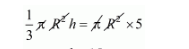# The material of a cone is converted into the shape`
Question:

The material of a cone is converted into the shape of a cylinder of equal radius. If height of the cylinder is 5 cm, then height of the cone is

(a) 10 cm

(b) 15 cm

(c) 18 cm

(d) 24 cm

Solution:

A cone is converted into a cone.

So,

Volume of cone = Volume of cylinder$h=15 \mathrm{~cm}$

Hence, the correct answer is choice (b).